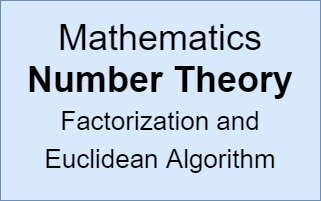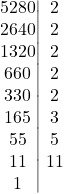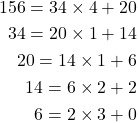# Mathematics - Number Theory - Factorization and Euclidean Algorithm

in STEMGeeks9 months ago## Introduction

Hey it's a me again @drifter1!

Today we continue with Mathematics, and more specifically the "Number Theory" series, in order to get into the Factorization and Euclidean Algorithm. The Euclidean algorithm is perhaps the most important algorithm in Number theory.

So, without further ado, let's get straight into it!

## Solution to Exercise

Let's check if 5280 is divisible by 2-to-12 by applying the divisibility rules of the previous article.

• 2 : yes as it's an even number (or last digit is 0).
• 3 : yes as 5 + 2 + 8 + 0 = 15 which is divisible by 3.
• 4 : yes as the number formed by the last two digits "80" is divisible by 4.
• 5 : yes as the last digit is 0.
• 6 : yes as both the 2-rule and 3-rule are satisfied.
• 7 : no as 528 - 0 × 2 → 52 - 8 × 2 = 36 which is not divisible by 7.
• 8 : yes as the number formed by the last three digits "280" is divisible by 8.
• 9 : no as 5 + 2 + 8 + 0 = 15 which is not divisible by 9.
• 10: yes as the number ends with a 0.
• 11: yes as 5 - 2 + 8 - 0 = 11 which is divisible by 11.
• 12: yes as number is divisible by both 3 and 4.

## Factorization

Any given number can be factorized based on the divisors and those are mostly only the prime divisors, as all numbers can be represented by primes and their combinations.

For example, 5280 can also be written as:

5280 = 25 × 3 × 5 × 11.

which has been computed as follows:It's now easier to see why the number is divisible by the previously proven numbers: 2, 3, 4, 5, 6, 8, 10, 11 and 12 if we take the numbers in corresponding pairs. Additionally, other possible divisors are for example 24 = 16, 25 = 32 or 2 × 11 = 22. So, a given number is divisible by every possible combination of its factors.

## Euclidean Algorithm and GCD

So, we now know how to check for the divisibility and have found a way to represent at least small numbers with ease based on their divisors.

Now the questions is:

Do we always have to factor the numbers or is there a way to check the common divisors between two numbers and maybe even of computing the greatest common divisor without having to factor the numbers?

That's when the Euclidean Algorithm comes into play...

The Euclidean Algorithm is a method for efficiently computing the greatest common divisor (GCD) of two integers.

The Euclidean algorithm goes as follows:

1. Let a and b be two integers.
2. Divide the numbers using the division algorithm: a = bq + r, where q is the quotient and r the remainder of the divison of a and b.
3. If r = 0 then b is the GCD of a and b.
4. Otherwise replace a and b with b and r and repeat step 2.

### Example

Let's calculate the GCD of 156 and 34.and so the GCD is 2.

If the GCD wasn't 2 and was a larger number then dividing the two numbers by that number and repeating the process would provide us with the next common divisor. Repeating the process only makes sense when the GCD is not 1 or 2.

## Division Algorithm using Calculator

How one proceeds with calculating the quotient and remainder is a matter of personal preference. The most commonly used division is the so called Euclidean division which is taught in most schools. Of course, this algorithm takes a lot of time when the numbers are large.

For larger numbers, and when speed is key, a procedure for getting the result to the necessary a = bq + r format is the following:

1. Divide the numbers a and b using a calculator which yields a number c. If the result is a whole number then b | a.
2. Round down c to the next whole number or simply remove the digits after the decimal point. The resulting number is the needed quotient q.
3. Multiply b and q and subtract the result from a. The result is the needed r.

### Example

Let's divide 17832 by 439.

1. Using a calculator the result is 17832 ÷ 439 = 40.6195899772
2. Thus, q = 40.
3. Lastly, 17832 - 439 × 40 = 17832 - 17560 = 272 = r.

So, 17832 = 439 × 40 + 272.

## RESOURCES:

### References

Block diagrams and other visualizations were made using draw.io.

## Final words | Next up

And this is actually it for today's post!

Next time we will probably get into Prime numbers...

See ya!Keep on drifting!

Posted with STEMGeeks

Sort:

Nice work drifter
Yeah we should keep drifting on😀

Your content has been voted as a part of Encouragement program. Keep up the good work!

Use Ecency daily to boost your growth on platform!

Support Ecency
Vote for new Proposal
Delegate HP and earn moreThis post was shared and voted inside the discord by the curators team of discovery-it
Discovery-it is also a Witness, vote for us here
Delegate to us for passive income. Check our 80% fee-back Program

Euclidean is a familiar term to me in sequencing generative music.#### You have received a 1UP from @lipe100dedos!

The @oneup-cartel will soon upvote you with:
@stem-curator
And they will bring !PIZZA 🍕.

Thank you so much for sharing the explanation.
!1UP

You can earn passive income by delegation of tribe tokens to "The Cartel".Click this banner to join "The Cartel" discord server to know more.

9 months ago

It’s always trippy for me to view numbers so differently from conventional thought process.

!discovery 37

9 months ago Reveal Comment